Updating search results...

# 111 Results

View
Selected filters:
• Area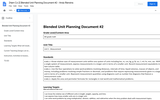Conditional Remix & Share Permitted
CC BY-NC-SA
Rating
3.0 stars
Overview:

This unit is basically chapter 5 in our Math Expressions curriculum.  If your district uses Math Expressions, then this unit will make a lot of sense to you.  If you don't have Math Expressions, this unit can still help guide you through teaching your kids meausurement using customary and metric systems.

Subject:
Mathematics
Material Type:
Unit of Study
Provider:
Michigan Virtual
Author:
Andy Rienstra
11/25/2016Conditional Remix & Share Permitted
CC BY-NC-SA
Rating
0.0 stars
Overview:

This is an introductory project-based lesson. It is assumed that background knowledge of vocabulary, procedural steps, and concept have been previously instructed.  In this project, students will create a square-based pyramid net that would represent their actual tent. Then, they will need to figure out how much fabric that they will need in order to cover their tent.  Extensions are built into this activity that would allow the students to practice / explore skills with decimals, percents, and ratios.

Subject:
Mathematics
Material Type:
Activity/Lab
Author:
William Allred
Carrie Robledo
05/06/2021Rating
0.0 stars
Overview:

Sample Item provided by Smarter Balanced as preliminary examples of the types of items that students might encounter on the summative assessment. The item assesses whether students can perform arithmetic operations on polynomials. MAT.HS.ER.3.0AAPR.F.045

Subject:
Math 1
Math 2
Material Type:
Activity/Lab
Assessment
Provider:
Oregon Department of Education
Author:
Smarter Balanced Assessment Consortium
02/26/2019Rating
4.0 stars
Overview:

A Constructed Response Item provided by Smarter Balanced as preliminary examples of the types of items that students might encounter on the summative assessment. The item prompt students to produce a text or numerical response in order to collect evidence about their knowledge or understanding of using structure of an expression to identify ways to rewrite it and rearrange formulas to highlight a specific quantity of interest. MAT.HS.CR.2.0ASSE.A.005

Subject:
Math 1
Math 3
Material Type:
Activity/Lab
Assessment
Provider:
Oregon Department of Education
Author:
Smarter Balanced Assessment Consortium
02/26/2019Rating
0.0 stars
Overview:

Students are given a scenario and must select three rectangles to show how they could use Jack's strategy of breaking apart a rectangle to find the area of each figure.

Subject:
Mathematics
Material Type:
Activity/Lab
Provider:
K-5 Math Teaching Resources
Author:
K-5 Math Teaching Resources
02/26/2019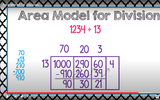Unrestricted Use
CC BY
Rating
0.0 stars
Overview:

This is one possible method for using the area model for division.

With this division strategy, students divide by breaking the dividend into its expanded form. Then, students use familiar multiplication facts to divide. It is suggested that this would be the first of three strategies for division (preceding partial quotients and the standard algorithm.

Subject:
Mathematics
Material Type:
Activity/Lab
Diagram/Illustration
Interactive
Teaching/Learning Strategy
Author:
Dawne Coker
06/28/2020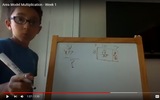Unrestricted Use
CC BY
Rating
0.0 stars
Overview:

In this video, a student explains how to use the area model for multiplication. The area model is a multiplication strategy based on decomposing numbers basedon place value.

Subject:
Mathematics
Material Type:
Activity/Lab
Diagram/Illustration
Interactive
Teaching/Learning Strategy
Author:
Dawne Coker
06/28/2020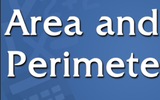Conditional Remix & Share Permitted
CC BY-NC-SA
Rating
0.0 stars
Overview:

This resource provides a multiday station-rotation blended learning lesson plan for teaching Area and Perimeter.

Subject:
Mathematics
Material Type:
Formative Assessment
Game
Lesson Plan
Teaching/Learning Strategy
Vocabulary
Author:
JOSEPH HAYES JR
06/24/2021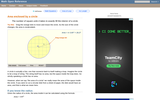Rating
0.0 stars
Overview:

An interactive applet and associated web page that demonstrate the area of a circle. A circle is shown with a point on the circumference that can be dragged to resize the circle. As the circle is resized, the radius and the area computation is shown changing in real time. The radius and formula can be hidden for class discussion. Applet can be enlarged to full screen size for use with a classroom projector. This resource is a component of the Math Open Reference Interactive Geometry textbook project at http://www.mathopenref.com.

Subject:
Geometry
Material Type:
Simulation
Provider:
Math Open Reference
Author:
John Page
05/07/2019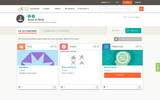Conditional Remix & Share Permitted
CC BY-NC-SA
Rating
1.0 stars
Overview:

This short video and interactive assessment activity is designed to teach third graders about area in grid.

Subject:
Mathematics
Material Type:
Assessment
Interactive
Lecture
Provider:
CK-12 Foundation
Provider Set:
CK-12 Elementary Math
06/06/2019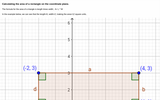Unrestricted Use
CC BY
Rating
2.0 stars
Overview:

Images of rectangle on coordinate plane, one with easily identifiable area, other image with same rectangle rotated. Distance formula shown to calculate same area.

Subject:
Geometry
Material Type:
Interactive
Provider:
Michigan Virtual
07/19/2019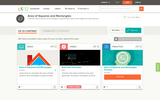Conditional Remix & Share Permitted
CC BY-NC-SA
Rating
2.5 stars
Overview:

This short video and interactive assessment activity is designed to teach third graders an overview of area of squares and rectangles - word problems.

Subject:
Mathematics
Material Type:
Assessment
Interactive
Lecture
Provider:
CK-12 Foundation
Provider Set:
CK-12 Elementary Math
06/06/2019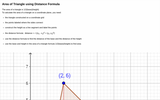Unrestricted Use
CC BY
Rating
1.66666666667 stars
Overview:

Definition of area of triangle, image of triangle on coordinate grid with height constructed. Area of triangle calculated using distance formula.

Subject:
Geometry
Material Type:
Interactive
Provider:
Michigan Virtual
07/19/2019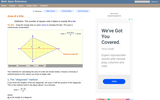Rating
5.0 stars
Overview:

An interactive applet and associated web page that deals with the area of a kite, (a quadrilateral with two distinct pairs of equal adjacent sides). The applet shows a kite and the user can reshape it by dragging any vertex. The other vertices move automatically to ensure it always remains a kite. As the vertices are dragged, the area is continuously recalculated and displayed. The kite is filled with a grid of unit squares so that the students can estimate the area. The on-screen calculation can be hidden until the estimates are done. The web page lists two different ways to compute the area of a kite. Applet can be enlarged to full screen size for use with a classroom projector. This resource is a component of the Math Open Reference Interactive Geometry textbook project at http://www.mathopenref.com.

Subject:
Geometry
Material Type:
Simulation
Provider:
Math Open Reference
Author:
John Page
05/07/2019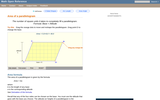Rating
0.0 stars
Overview:

A web page and interactive applet showing the ways to calculate the area of a parallelogram. The user can drag the vertices of the parallelogram and the other points change automatically to ensure it remains a parallelogram. A grid inside the shape allows students to estimate the area visually, then check against the actual computed area, which is continuously recomputed and displayed. The text on the page gives three different ways to calculate the area with a formula for each. The applet uses one of the methods to compute the area in real time, so it changes as the rhombus is reshaped with the mouse. A companion page is http://www.mathopenref.com/parallelogram.html showing the definition and properties of a parallelogram Applet can be enlarged to full screen size for use with a classroom projector. This resource is a component of the Math Open Reference Interactive Geometry textbook project at http://www.mathopenref.com.

Subject:
Geometry
Material Type:
Simulation
Provider:
Math Open Reference
Author:
John Page
05/07/2019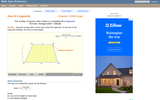Rating
0.0 stars
Overview:

A web page and interactive applet showing the ways to calculate the area of a trapezoid. The user can drag the vertices of the trapezoid and the other points change automatically to ensure it remains a trapezoid. A grid inside the shape allows students to estimate the area visually, then check against the actual computed area. The text on the page gives three different ways to calculate the area with a formula for each. The applet uses one of the methods to compute the area in real time, so it changes as the trapezoid is reshaped with the mouse. A companion page is http://www.mathopenref.com/trapezoid.html showing the definition and properties of a trapezoid. Applet can be enlarged to full screen size for use with a classroom projector. This resource is a component of the Math Open Reference Interactive Geometry textbook project at http://www.mathopenref.com.

Subject:
Geometry
Material Type:
Simulation
Provider:
Math Open Reference
Author:
John Page
05/07/2019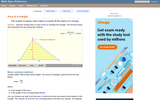Rating
5.0 stars
Overview:

An interactive applet and associated web page that explain the area of a triangle. The applet shows a triangle that can be reshaped by dragging any vertex. As it changes, the area is continually recalculated using the 'half base times height' method. The triangle has a fixed square grid in its interior that can be used to visually estimate the area for later correlation with the calculated value. The calculation can be hidden while estimation is in progress. The text page has links to a similar page that uses Heron's Formula to compute the area. Applet can be enlarged to full screen size for use with a classroom projector. This resource is a component of the Math Open Reference Interactive Geometry textbook project at http://www.mathopenref.com.

Subject:
Geometry
Material Type:
Simulation
Provider:
Math Open Reference
Author:
John Page
05/07/2019Conditional Remix & Share Permitted
CC BY-NC-SA
Rating
0.0 stars
Overview:

This book is a "flexed" version of CK-12's Basic Geometry that aligns with College Access Geometry and contains embedded literacy supports. It covers the essentials of geometry for the high school student.

Subject:
Mathematics
Material Type:
Textbook
Provider:
CK-12 Foundation
Provider Set:
CK-12 FlexBook
Author:
Fauteux, Michael
Zapata, Rosamaria
05/18/2011Conditional Remix & Share Permitted
CC BY-NC-SA
Rating
4.0 stars
Overview:

CK-12 Foundation's Geometry FlexBook is a clear presentation of the essentials of geometry for the high school student. Topics include: Proof, Congruent Triangles, Quadrilaterals, Similarity, Perimeter & Area, Volume, and Transformations.

Subject:
Mathematics
Material Type:
Textbook
Provider:
CK-12 Foundation
Provider Set:
CK-12 FlexBook
10/06/2009Rating
0.0 stars
Overview:

In this activity, the students will demonstrate the ability to represent numbers in scientific notation and use geometry to solve problems about planets in the solar system.

Subject:
Mathematics
Material Type:
Activity/Lab
Lesson Plan
Provider:
PBS
Author:
PBS Mathline